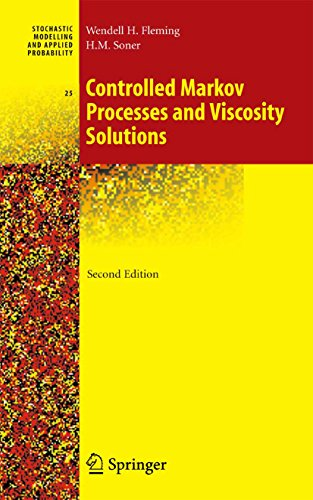Controlled Markov Processes and Viscosity Solutions

Wendell H. Fleming H. Mete Soner

Yayınevinden satın almak için tıklayınız.

This book is intended as an introduction to optimal stochastic control for continuous time Markov processes and to the theory of viscosity solutions. Stochastic control problems are treated using the dynamic programming approach. The authors approach stochastic control problems by the method of dynamic programming. The fundamental equation of dynamic programming is a nonlinear evolution equation for the value function. For controlled Markov diffusion processes, this becomes a nonlinear partial differential equation of second order, called a Hamilton-Jacobi-Bellman (HJB) equation. Typically, the value function is not smooth enough to satisfy the HJB equation in a classical sense. Viscosity solutions provide framework in which to study HJB equations, and to prove continuous dependence of solutions on problem data. The theory is illustrated by applications from engineering, management science, and financial economics.

In this second edition, new material on applications to mathematical finance has been added. Concise introductions to risk-sensitive control theory, nonlinear H-infinity control and differential games are also included.

Review of the earlier edition:

“This book is highly recommended to anyone who wishes to learn the dinamic principle applied to optimal stochastic control for diffusion processes. Without any doubt, this is a fine book and most likely it is going to become a classic on the area… .”

SIAM Review, 1994

Yılı: 2006
Yayınevi: Springer
ISBN: 978-1-4419-2078-2

*Springer WEB Sitesi’nden alınmıştır.